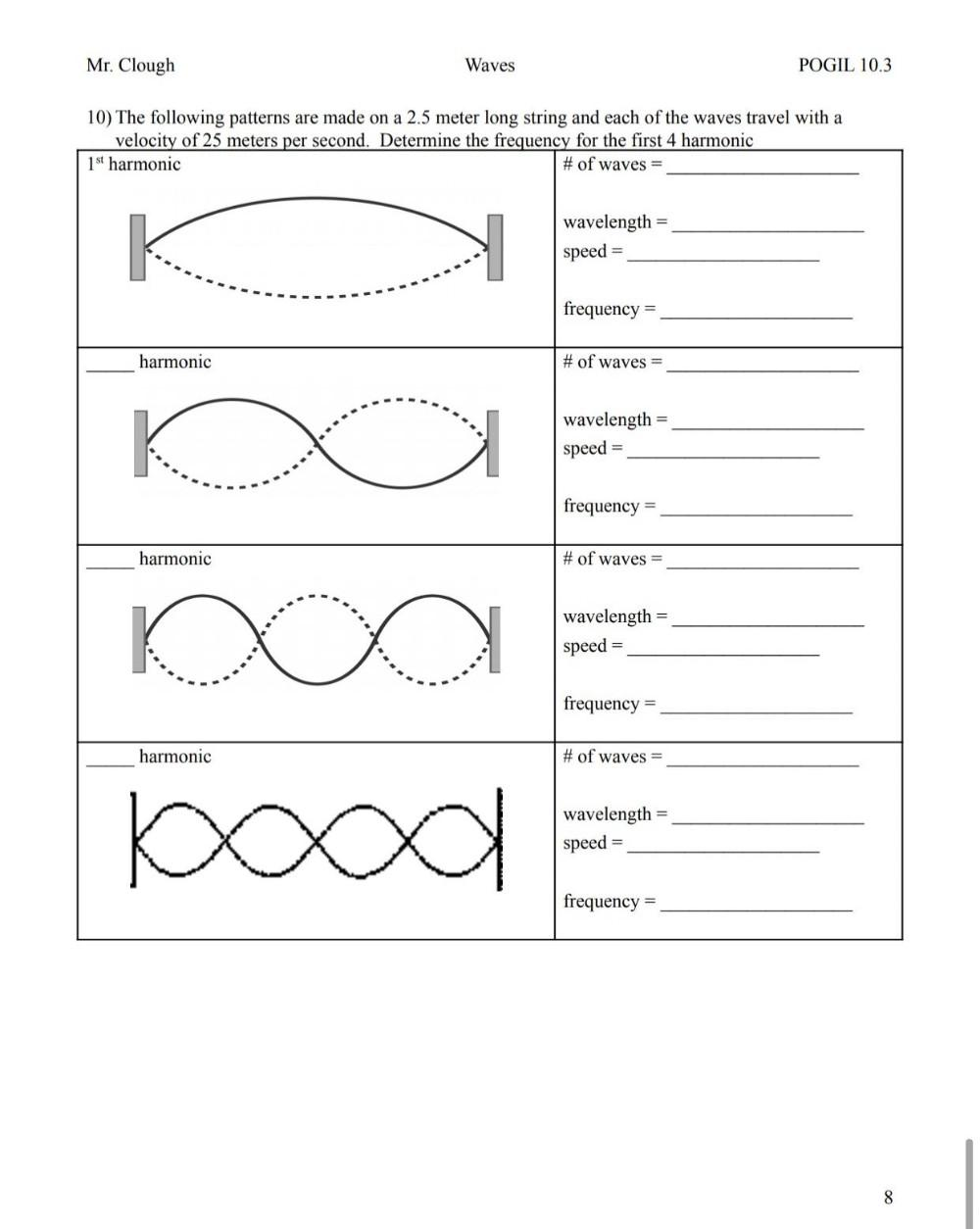Question:

# Mr. Clough Waves POGIL 10.3 10) The following patterns are made on a 2.5 meter long string and each of the waves travel with a vMr. Clough Waves POGIL 10.3 10) The following patterns are made on a 2.5 meter long string and each of the waves travel with a velocity of 25 meters per second. Determine the frequency for the first 4 harmonic 1st harmonic # of waves = wavelength speed frequency = harmonic # of waves = K wavelength speed frequency = harmonic # of waves = ko O wavelength speed = frequency = harmonic # of waves = ba wavelength speed frequency = 8